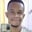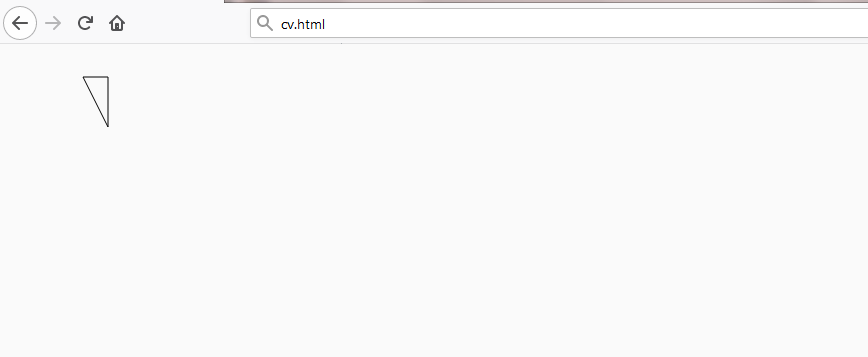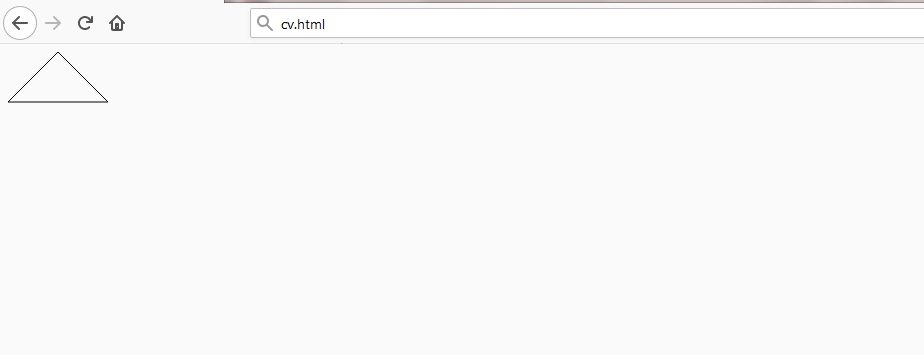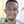# How to draw triangles in HTML CanvasChidume Nnamdi

### Overview

canvas doesn’t have dedicated APIs to draw triangles, so we can use the lineTo and moveTo methods to create them.

const context = canvas.getContext("2d");
context.beginPath()
context.moveTo(75, 25)
context.lineTo(100, 75)
context.lineTo(100, 25)
context.lineTo(75, 25)
context.stroke()
context.closePath()


The code above will create a right-angled triangle.

### Explanation

• moveTo(75,25) sets the pen at $x,y$ = $(75,25)$.

• lineTo(100,75) marks a line from $x,y$ = $(75,25)$ to $x,y$ $(100,75)$.

• lineTo(100,25) marks a line from the previous endpoint, $x,y$ = $(100,25)$.

• lineTo(75, 25) marks a line from $x,y$ = $100,25$ to $(x,y)$ $(72,25)$. This marks the right-angle.

• Finally, stroke() creates the outline.Now, let’s create a regular triangle:

context.beginPath()
context.moveTo(0, 50)
context.lineTo(50, 0)
context.lineTo(100, 50)
context.lineTo(0, 50)
context.stroke()
context.closePath()


The command above will produce the following:Note: Please refer to the following shots for information related to this topic:
-Drawing lines and paths
-Filling paths
-Drawing rectangles
-Drawing circles and arcs

RELATED TAGS

javascript
node

CONTRIBUTORChidume Nnamdi